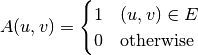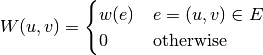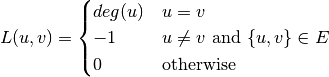# Matrix Representation¶

Matrix representation of graphs are widely used in algebraic analysis of graphs. This package comprises functions that derive matrix representation of an input graph.

An adjacency matrix is defined asadjacency_matrix(graph)

Constructs an adjacency matrix for a graph.

## Weight Matrix¶

A weight matrix is defined asweight_matrix(graph, eweights)

Constructs a weight matrix from a graph and a vector of edge weights. Here, g must implement edge_map and (edge_list or incidence_list).

## Distance Matrix¶

A distance matrix is defined asdistance_matrix(graph, eweights)

Constructs a distance matrix from a graph and a vector of edge weights. Here, g must implement edge_map and (edge_list or incidence_list).

## Laplacian Matrix¶

Laplacian matrix is significant in algebraic graph theory. The eigenvalues of a Laplacian matrix characterizes important properties of a graph. For an undirected graph, it is defined as:laplacian_matrix(graph)

Constructs a Laplacian matrix over an undirected graph.

For graphs with weighted edges, we have

laplacian_matrix(graph, eweights)

Constructs a weighted Laplacian matrix from an undirected graph with a vector of edge weights.